## May 06, 2006

### 5/3\$B!A(B5/5\$B\$NIw7J(B [\$B\$=\$NB>(B]

 \$B@6@!DL\$j!AM-L@J}LL(B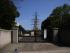\$BL@<#4](B \$BEl5~3\$MNBg3XFb(B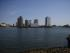\$BAj@866\$+\$i(B \$BK-='J}8~(B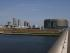\$BB?J,@5LL\$OF|K\%f%K%7%9(B \$B=U3\$66(B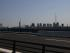\$B66\$N8~\$\$B&(B \$BM-L@J}8~\$@\$H;W\$&(B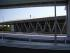\$BM-L@%3%m%7%"%`(B _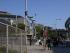\$B9q:]E8<(>lJ}8~(B \$B2?\$d\$C\$F\$?\$+\$OCN\$i\$s(B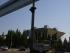\$B9q:]E8<(>l(B \$B%P%i%s%90-\$\$\$M(B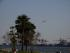\$B\$*Bf>l3\$IM8x1`(B \$B@N2?\$b\$J\$\$:"\$O\$h\$/Mh\$F\$\$\$?(B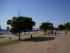\$B@N\$O%O%<\$,\$h\$/D`\$l\$?(B \$B:#\$OCN\$i\$s(B
 \$B3k@>NW3\$8x1`(B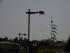\$B3\$IMKkD%\$N1XA0\$+\$i(B \$B5(@a\$NIwJ*;m(B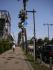\$B1XA0\$NDL\$j(B \$B\$J\$s\$@\$+\$J!<(B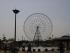\$B4QMw \$B9=\$o\$:FM\$C9~\$`;R6!(B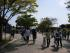\$B8x1`\$NCf(B \$B?M\$,B?\$\$(B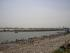\$B8~\$+\$\$\$O?M8}=m(B \$B\$=\$l\$J\$j(B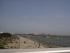\$BIqIMJ}8~(B \$B\$=\$l\$J\$j(B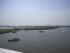\$B7k9=?eO)\$KA%\$,(B ...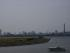\$BET?4J}8~\$rK>\$`(B \$B0F30;k3&\$O\$h\$/\$J\$\$(B?
Posted at 19:44 in \$B\$=\$NB>(B

### \$B%I%-%e%a%s%H(B [\$BK\(B,\$B;(;o\$J\$I(B]

• \$B?y;3(B \$BN4CK!VJ<;N\$r8+\$h!W(B(\$B?7D,

\$B<+1RBb%k%]%7%j!<%:\$N9R6u<+1RBbJT!#(B \$B\$3\$l\$rFI\$s\$GEbFM\$K!V%i%\$%H%9%?%C%U!W\$r8+\$?\$/\$J\$C\$?!#(B

### Video \$B!V%i%\$%H%9%?%C%U!W(B [\$B\$=\$NB>(B]

• \$B%i%\$%H%9%?%C%U(B \$B%9%Z%7%c%k%(%G%#%7%g%s(B(\$B%o!<%J!

\$B\$H\$\$\$&\$o\$1\$G(B(\$BK\!";(;o\$N9`;2>H(B)\$BGc\$C\$?!#K\JT(B193\$BJ,!"FCE51GA|(B169\$BJ,!#0B\$\$(B...

Posted at 00:20 in \$B\$=\$NB>(B